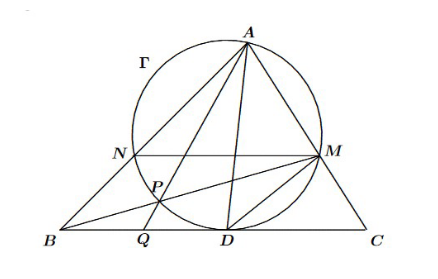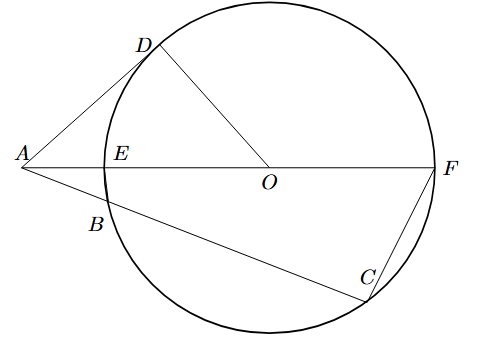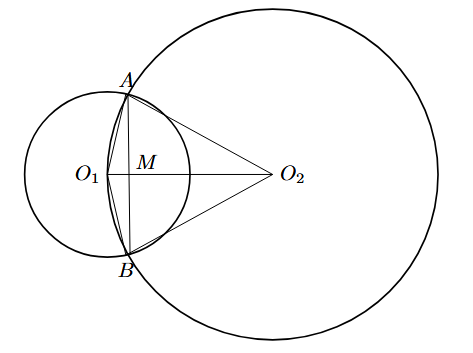Year:Nicolae Strungaru

## Problem of the Week

by Prof. Nicolae Strungaru, Grant MacEwan University

The CMS is extremely grateful to Dr. Nicolae Strungaru (MacEwan) for creating the Problems of the Week to help thousands of students prepare for the COMC each year. Thank you, Professor Strungaru!

The COMC has three parts. In part A solutions do not require work be shown and may be possible to do in your head. In part B the problems begin to draw on more knowledge and have some more challenging aspects that will need a pencil and paper to solve. By Part C the problems require that work be shown and involve arguments to support the answer.

We have selected a cross section of contest problems from a variety of national and regional contests that we hope will stimulate interest in problem solving and give some experience to get ready to write the Canadian Open Mathematics Challenge in October. The problem areas are not tied to particular grade levels, or to the curriculum but cover a number of areas from algebra, through logic and some geometry.

We will post solutions to these problems one week later, but teachers should be aware that determined students may be able to locate solutions elsewhere online before then.

For a more comprehensive set of problems and solutions at each of these levels, please feel welcome to download past official exams and solutions from our archive.

Week 8

• Problem (posted October 17th)

Find all pairs $(x,y)$ of positive integers such that $x^y = y^{x-y} \,.$

• Solution (posted October 24th)

Problem 3 from the 1998 Junior Balkan Mathematical Olympiad, which appeared in Crux Mathematicorum [2002:522]. We present the solution official solution that appeared in Crux at [2004:159], slightly modified by the editor.

If $x=1$ then we immediately get $y=1$.
Next, let us look at $x \geq 2$. In this case $y^{x-y}=x^y \geq 2^1$ which gives $y \geq 2$ and $x-y > 0$. In particular $x > y$. Therefore $y^{x-y}=x^y \geq y^y \Rightarrow x-y > y \Rightarrow x > 2y \,.$ This allows us rewrite the equation \begin{align} x^y&= y^{y}y^{x-2y} \Rightarrow \\ \cr (\frac{x}{y})^y &=y^{x-2y} \,. \cr \end{align} This shows that $(\frac{x}{y})^y$ is an integer. We claim that this implies that $y$ divides $x$. Indeed, write $\frac{x}{y}=\frac{a}{b}$ in the reduced form. Let us assume by contradiction that $b \neq 1$, then, our equation can be rewritten as $y^{x-2y}b^y=a^y \,.$ Now, let $p$ be any prime dividing $b$. Such prime exists since $b >1$. Then, $p$ must divide $y^{x-2y}b^y=a^y$ and hence $p$ divides $a$. But this contradicts the fact that $\frac{a}{b}$ is reduced.
Since we got a contradiction, our assumption that $b \neq 1$ is wrong.
It follows that $y$ divides $x$ and hence $x=ky$ for some positive integer $k$. Moreover, $x >2y$ gives $k >2$.
Our equation then becomes \begin{equation}\tag{6} k^y=y^{(k-2)y} \Rightarrow k =y^{k-2} \,. \end{equation} In particular, this implies that \begin{equation}\tag{7} k=y^{k-2} \geq 2^{k-2} \,. \end{equation} We claim that for $k \geq 5$ we have $2^{k-2}>k$. This can be proven by induction.
$P(5)$: $2^3=8 >5$.
$P(k)\Rightarrow P(k+1)$: $2^{k-1}=2\cdot 2^{k-2}> 2k > k+1$.
This completes the proof of the induction step.
This claim together with (6) implies that $k \leq 4$. Since $k>2$ we only have two possible values of $k$. We test them.
If $k=3$, equation (6) becomes $3=y^1$ giving the solution $y=3, x=ky=9$.
If $k=4$, equation (6) becomes $4=y^2$ giving the solution $y=2, x=ky=8$.
This shows that there are three solutions $(x,y) \in \{ (1,1) ; (8,2) ; (9,3) \} \,.$

Week 7

• Problem (posted October 10th)

This week we look at a geometry problem.

In triangle $\Delta ABC$, the angle bisector of $\angle BAC$ meets $BC$ at point $D$. Let $\Gamma$ be the circle which is tangent to $BC$ at $D$ and passes through the point $A$. Let $M$ be the second point of intersection of $\Gamma$ and $AC$ and let $P$ be the second point of intersection of $BM$ and $\Gamma$. Prove that $AP$ is the median of the triangle $\Delta ABD$.

• Solution (posted October 17th)

Problem 5 from the second round of the 19998-1999 Iranian Mathematical Olympiad, which appeared in Crux Mathematicorum [2001:486]. We present the solution by Miguel Amengual which appeared at [2004:159].

Let $N$ be the second point of intersection of $\Gamma$ and $AB$ and let $AP$ meet $BC$ at $Q$.Then $\angle MDC=\frac{\overset{\large \frown}{MD}}{2}=\angle CAD = \frac{1}{2} \angle A \,.$ We therefore get \begin{align} \angle ADM &=\angle ADC-\angle MDC \\ \cr &=(180^\circ-\angle CAD - \angle ACD)- \angle MDC \\ \cr &=180^\circ-\frac{1}{2}\angle A - \angle C- \frac{1}{2}\angle A =\angle B \,. \cr \end{align} Also, we have $\angle ADM=\frac{\overset{\large \frown}{AM}}{2}= \angle ANM= \angle APM \,.$ This shows that $\angle B=\angle ANM$ (which also shows that $MN \parallel BC$, but we will not need this).

We thus get $\angle B= \angle APM =\angle ANM =\angle QPB \,.$ This shows that \begin{align} \angle QPB &=\angle ABQ \\ \cr \angle BQP &= \angle BQA \cr \end{align} and hence the triangles $BQP$ and $AQP$ are similar. In particular $\frac{BQ}{AQ}=\frac{PQ}{BQ}$ giving $BQ^2=QP \cdot QA \,.$ Next, by the power of the point $Q$ with respect to $\Gamma$ (see editor's note below) we have $QP \cdot QA = QD^2 \,.$ This shows that $BQ=QD$, and hence that $AP$ is the median of the triangle.

Editor's note: Let us briefly review here the power of the point with respect to a circle. Let $\Gamma$ be the circle of radius $R$ with centre at $O$, let $A$ be a point outside the circle. Let $l_1$ be a line through $A$ that intersects $\Gamma$ at $B$ and $C$ and let $l_2$ be a line through $A$ which is tangent to the circle at $D$.

Then, we have $AB \cdot AC = AD^2 \,.$ In particular, $AB \cdot AC$ is independent of the choice of $l_1$. The constant $AB \cdot AC$ is called the power of $A$ with respect to $\Gamma$, and can be shown to be equal to $AO^2-R^2$.

Indeed, let $E,F$ be the two points of intersection of the line $AO$ with $\Gamma$.Indeed, since $AD \perp OD$, by Pythagoras' theorem we have $AD^2=AO^2-R^2 \,.$ Next, $\angle AEB= 180^\circ -\angle BEF =180^\circ - \frac{\overset{\large \frown}{BCF}}{2}=\frac{\overset{\large \frown}{BEF}}{2}=\angle BCF \,.$ By the similarity of the triangles $AEB$ and $ACF$ we get $AB \cdot AC= AE \cdot AF = (AO-R)(AO+R)=AO^2-R^2 \,.$ This establishes the power of the point formula.

Week 6

• Problem (posted October 3rd)

Find all positive integers $m,n$ such that $2^n+n=m! \,.$

• Solution (posted October 10th)

Problem 1 from day 2 of the 2013 Turkey Mathematical Olympiad, which appeared in Crux Mathematicorum [2014:375]. We present the solution by Oliver Geupel which appeared at [2016:14-15], slightly modified.

Write $n=2^k\cdot l$ for some non-negative integer $k$ and an odd number $l$.

Then, $m!=2^{2^k\cdot l}+2^k\cdot l=2^k \left(2^{2^k \cdot l-k}-l \right) \,.$ Since $2^k \cdot l>k$ and $l$ is odd, it follows that the power of $2$ in $m!$ is $k$. This implies that there are at most $k$ even numbers up to $m$, and hence $m \leq 2k+1\,.$ Then \begin{equation}\tag{5} 2^{2^k} \leq 2^{2^k\cdot l}+2^k\cdot l = m! \leq (2k+1)! \,. \end{equation} We claim that for $k \geq 5$ we have $2^{2^k} >(2k+1)!$. We prove this by mathematical induction.

$P(5)$: $11!=2^8\cdot 3^4 \cdot 5^2 \cdot 7 \cdot 11 < 2^{8} \cdot 2^{7} \cdot 2^5 \cdot 2^3 \cdot 2^4=2^{27} <2^{32} =2^{2^5} \,.$ $P(k)\Rightarrow P(k+1)$: We have \begin{align} 2^{2^{2k+1}}&=(2^{2^k})^2 > ((2k+1)!)^2 > (2k+1)! \cdot \left(2 \cdot (2k+1) \cdot 3 \cdot (2k) \right)\\ \cr &> (2k+1)!(2k+2)(2k+3)=(2k+3)! \,. \cr \end{align}

This completes the induction.

Now, (5) and our claim give $k \leq 4$ and hence $m \leq 2k+1 \leq 9$.

Testing all possibilities $1 \leq m \leq 9$, we see that the only solution is $(n,m)=(2,3)$.

Week 5

• Problem (posted September 26th)

Find all the functions $f$ from the set of real numbers to the set of real numbers which satisfy \begin{equation}\tag{2} f(y-xy)=f(x)y+(x-1)^2f(y) \end{equation} for all real numbers $x,y$.

• Solution (posted October 3rd)

Problem 3 of the 2017 Czech-Slovakia Math Olympiad, which appeared in Crux Mathematicorum [2019:17]. We present the solution by Sundara Narasimhan that appeared at [2019:397-398], modified by the editor.

Setting $x=1$ in (2) we get that for all real numbers $y$ we have $f(0)=f(1)\cdot y \,.$ Since the right hand side is constant, so is the left hand side and therefore $f(0)=f(1)=0 \,.$ Now, setting $y=1$ in (2) we get that for all real numbers $x$ we have $f(1-x)=f(x) \,.$ Next, let $t$ be a real number. Setting $x=1-t$ in (2) and using $f(1-t)=f(t)$ we get \begin{equation}\tag{3} f(ty)=f(t)y+t^2f(y) \end{equation} for all real numbers $t,y$.

Interchanging $t,y$ in (3) we get \begin{equation}\tag{4} f(ty)=f(y)t+y^2f(t) \end{equation} and therefore, for all real numbers $y,t$ we have $f(y)t+y^2f(t)=f(t)y+t^2f(y) \Rightarrow (y^2-y)f(t)=(t^2-t)f(y) \,.$ Setting $y=2$ in this last relation, we get that for all real numbers $t$ we have $f(t)=\frac{f(2)}{2}(t^2-t)=c(t^2-t) \,,$ where $c=\frac{f(2)}{2}$ is a real number. Therefore, all potential solutions must have the form $f(t)=c(t^2-t) \,.$ Let us now check which such functions are solution.

Plugging $f(x)=c(x^2-x)$ into (2) we get \begin{align} c\left((y-xy)^2-(y-xy)\right) &=c(x^2-x)y+(x-1)^2c(y^2-y) \Leftrightarrow \\ \cr c\left(y^2-2xy^2+x^2y^2-y+xy\right) &=c(x-1)y \left(x+(x-1)(y-1)\right)\Leftrightarrow \\ \cr cy\left(y-2xy+x^2y-1+x\right) &=cy(x-1) \left(xy-y+1\right)\Leftrightarrow \\ \cr cy\left(y-2xy+x^2y-1+x\right) &=cy \left(x^2y-2xy+x+y-1\right) \checkmark \cr \end{align} This shows that all $f(x)=c(x^2-x)$ are solutions.

Therefore, the functions satisfying the given relation are

$f(x)=c(x^2-x)$ where $c$ is a real number.

Editor's note: This type of problem is typically called a functional equation. A very common mistake when solving any functional equation is not checking that the potential solutions are actually solutions, which is typically easy but necessary.

Week 4

• Problem (posted September 19th)

Let $n$ be a positive integer. Show that $n \cdot (n+1) \cdot (n+2)$ is not a perfect square.

• Solution (posted September 26th)

Problem 4 from the 2005 Thai Mathematical Olympiad, which appeared in Crux Mathematicorum [2009:22]. We present the solution by Amengual Covas which appeared at [2010:156], slightly modified.

Note first that \begin{equation}\tag{1} n(n+2)=n^2+2n=(n+1)^2-1 \,. \end{equation} This immediately implies that $\mbox{gcd} (n+1, n(n+2))=1$. Indeed, $\mbox{gcd} (n+1, n(n+2))$ must divide both $n(n+2)$ and $(n+1)^2$ and hence , it must divide their difference $(n+1)^2-n(n+2)=1 \,.$ Let us now assume by contradiction that $n \cdot (n+1) \cdot (n+2)$ is a perfect square. Since $n+1$ and $n(n+2)$ are relatively prime, it follows that both $n+1$ and $n(n+2)$ must be perfect squares. Therefore, there exist non-negative integers $m,k$ such that \begin{align} n+1 &=k^2 \\ \cr n(n+2) &=m^2 \,. \cr \end{align} Plugging this into (1), we get $m^2=k^4-1 \Rightarrow (k^2-m)(k^2+m)=1 \,.$ Since $k^2+m \geq 0$ we get $k^2+m=k^2-m=1$ and hence $k=1, m=0$, which gives $n=0$. But this contradicts the fact that $n$ is a positive integer.

Since we got a contradiction, our assumption that $n \cdot (n+1) \cdot (n+2)$ is a perfect square is wrong.

Week 3

• Problem (posted September 12th)

Find all the values of $a$ such that the zeroes of the polynomial $f(x)=x^2-ax+2a$ are integer.

• Solution (posted September 19th)

Problem 21 from the 2015 level 4 phase 2 of the Primavera contest in Spain, which appeared in Crux Mathematicorum [2015:192], slightly modified. We present the solution by Henry Ricardo which appeared at [2016:199].

Let $r_1,r_2$ be the two roots. Then $x^2-ax+2a=(x-r_1)(x-r_2)$ gives \begin{align} r_1+r_2 &=a \\ \cr r_1r_2 &=2a \,, \cr \end{align} and hence $r_1r_2=2(r_1+r_2) \,.$ It follows that $r_1r_2-2r_1-2r_2=0$ and thus $(r_1-2)(r_2-2)=r_1r_2-2r_1-2r_2+4=4 \,.$ There are only four ways to write $4$ as product of integers: $(\pm 1) \cdot (\pm 4)$ and $(\pm 2)\cdot (\pm 2)$.

The four possibilities yield the following four pairs of solutions : $(0,0), (1,-2), (3,6)$ and $(4,4)$ giving $a \in \{ -1,0,8,9 \} \,.$

Editor's note: If $r_1,r_2$ are the roots of $ax^2+bx+c=0$ we have \begin{align} r_1+r_2 &=-\frac{b}{a} \\ \cr r_1r_2 &=\frac{c}{a} \,. \cr \end{align} These relations are called the Vieta's formulas, and there exists similar Vieta's formulas for polynomials of degree $n$, relating the roots to the coefficients of the polynomial.

Week 2

• Problem (posted September 5th)

If $x,y,z$ are real numbers so that \begin{align} x &=\sqrt{11-2yz} \\ \cr y &=\sqrt{12-2xz} \\ \cr z&=\sqrt{13-2xy} \,. \cr \end{align} find $x+y+z$.

• Solution (posted September 12th)

Problem 7 from the Mathematics Association of Quebec Contest, Secondary level, 2011, which appeared in Crux at [2011:483]. We present the solution by Richard I. Hess which appeared at [2013:8], slightly modified.

Squaring the three relations we get \begin{align} x^2+2xy &=11 \\ \cr y^2+2xz &=12 \\ \cr z^2+2yz &=13 \,. \cr \end{align} Adding these relations together we get $(x+y+z)^2=x^2+y^2+z^2+2xy+2xz+2yz=36 \,.$ Therefore, $x+y+z=\pm 6$.

Since $x,y,z$ are square roots, they are non-negative, and hence $x+y+z \geq 0$. This proves that $x+y+z=6 \,.$

Week 1

• Problem (posted August 29th)

We give two entry level problems this week. Give them a try. Look for the source and the solution next week!

Problem A
In how many ways is it possible to choose four distinct integers from $\{ 1; 2; 3; 4; 5; 6 ; 7 \}$, so that their sum is even?

Problem B
Two circles, one of radius 1, the other of radius 2, intersect so that the larger circle passes through the centre of the smaller circle. Find the distance between the two points at which the circles intersect.

• Solution (posted September 5th)

Problem A

Problem A5 of the 1998 Descartes Contest, which appeared in Crux at [2017:376]. We present the solution by Kathleen E. Lewis that appeared at [2018:369].

ince there are four odd numbers and three even numbers, to get an even sum we must have either four odd numbers, or two odd numbers and two even numbers. We can chose four odd numbers in $1$ way. We can choose two odd numbers and two even numbers in $\binom{4}{2}\cdot \binom{3}{2}=18$ ways.

Therefore there are $19$ ways of choosing four numbers so that their sum is even.

Editor's note: Try to solve the more general problem: in how many ways can we choose four distinct integers from $\{1,2,3,\ldots, n\}$ so that their sum is even?

What if we choose $k$ distinct integers from $\{1,2,3,\ldots, n\}$ for some $k \leq n$?

Problem B

Problem CC203 that appeared in Crux at [2016:4], whose solution appeared at [2017:9]. We present an alternate solution by the editor.Let $M$ be the midpoint of $AB$. Since the triangle $O_1AB$ is isosceles we have $O_1M \perp AB$. Same way, $O_2M \perp AB$. This shows that $O_1,M,O_2$ are collinear.

By Pythagoras theorem applied in $\Delta O_1MA$ and $\Delta O_2MA$ we have

\begin{align} O_2M^2+MA^2 &=O_2A^2=4 \,, \cr O_1M^2+MA^2 &=O_1A^2=1 \,. \cr \end{align}

Using $O_2M+O_1M=O_2O_1=2$ and subtracting the two relations we get \begin{align} 3 &=O_2M^2-O_1M^2=(O_2M-O_1M)(O_2M+O_1M) \cr &=2(O_2M-O_1M) \,. \cr \end{align} This gives $O_2M-O_1M=\frac{3}{2}$. Since $O_2M+O_1M=2$ we get $O_1M=\frac{1}{4}$ and hence \begin{align} MA^2=O_1A^2-O_1M^2=\frac{15}{16} \,. \cr \end{align} Then \begin{align} AB=2MA=\frac{\sqrt{15}}{2} \,. \cr \end{align}

Editor's note: Try to solve the same problem where the two circles have radiuses $r$ and $R$.